CHE4: HEATS OF CHEMICAL REACTIONS

This unit outlines the variant effects heat has on different chemical elements

It’s a study of heat changes accompanying chemical reactions

Exothermic reactions

Are those which precede with evolution of heat energy o the surroundings e.g. burning of substances like charcoal on the charcoal stove?

Exothermic reactions involve a rise in temperature

They involve a decrease in the energy content of the system i.e;-

-Energy of the products is less than energy of the reactants.

-Exothermic reactions are accompanied by temperature rise.

Endothermic reactions

These involve absorption of heat energy from the surroundings. They are associated with a temperature fall. An endothermic reaction involves an increase in the energy content of the system i.e. energy of products is greater than energy of reactions.

Dissolving of ammonium nitrate in water is an example of an endothermic reaction

Unit of heat change

Volts × coulombs = Joules

1kgJ   = 1000 J

The ΔH notation

Δ means change in Δ H is change in beat content or energy connect of a system.

Delta it is an always written in connection with a chemical equation representing the reaction to which the energy changes is associated.

Note:

Unless otherwise stated, the magnitude of delta it corresponds to mole reacting quantities as expressed in the reactions equations. By conversion, Δ H is given a negative sign in exothermic reactions and a positive sign for endothermic reactions.

Examples of exothermic chemical reactionsInterpretation of the equations

Equation 1

When one mole of solid carbon combines with one mole of gaseous oxygen to give one mole of gaseous carbon dioxide the heat liberated is 406 KJ.

Equation 2

When one mole of hydrogen gas reacts with ½ a mole of oxygen to give one mole of liquid water the heat liberated is 286 KJ.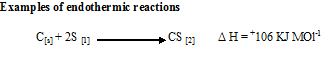When one mole of solid carbon combines with 2 moles of sulphur to give one mole of carbon disulphide heat observed is 106 KJ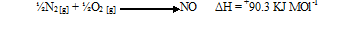When half a mole of Nitrogen gas combines with ½ amle of oxygen gas to give mole of nitrogen heat observed is 90.3KJ.

ΔHQ

Is heat change measured under standard condition of temp and pressure?

Standard temp-298 k =25 0C

Standard pressure- 760 mm Hg

Types of heat change

• Heat of combustion

An experiment to determine heat of combustion of ethanol or any other liquid fuel

It is always negative (is, Ts exothermic and heat is always given off when burnt in oxygen.

Heat of combustion is an important quantity used for industrial and domestic sources of energy concerning burning material.

Definition

Heat  of combustion is the heat change which taxes place when one mole substance completely burns in oxygen among their quantities good fuel mst burn to liberate a lot of heat energy per unit mass.

Enthalpy of solution

The enthalpy of solutionenthalpy of dissolution, or heat of solution is the enthalpy change associated with the dissolution of a substance in a solvent at constant pressure resulting in infinite dilution. The enthalpy of solution is most often expressed in kJ/mol at constant temperature.

Heat precipitation

To measure the heat of precipitation of silver chloride

Use a measuring cylinder to add 25cm3 of 0.5 m silver nitrate solution to a plastic bottle or cup, of about 70cm capacity. Rinse the measuring cylinder with water and then add 25cm3 of 0.5 silver nitrate solution to a plastic bottles or cup, of about 70 cm3 capacities. Rinse the measuring cylinder with water and then add 23cm3 of 0.5M solution cadium chloride (or potassium chloride or ammonium chloride) to it.

Both solutions should be @ room temperature Measure the temperature of the chloride solution, preferably using a thermometer graduated to 0.10C .Add the chloride solution to the silver nitrate. Shake well, and they measure the highest temperature be t0c (it is only a few degree).

If 500 cm3 of each solution had been used, the temperature rise would still be t0c because the heat change is 20times greater than with only 25cm3 but there is 20 times more product to heat (however, heat losses to the plastic container and the air are not so important when larger volume, are used and the results with more accurate) If the concentrations of solutions had been 2.0M instead of 0.5M, clearly the temperature.

ELECTRO CHEMICAL CELLSDevice for converting energy of chemical reaction in to electrical energy. Galvani or voltaic. The common galvanic or voltaic sell is that of zinc and copper strips deeper in to 2M sulphuric acid and dipped in connected to a voltmeter by wire.

The voltmeter indicate the voltage of 1.0 the zinc being more electro positive  than copper losses two electronics to form zn 2+ which enter the solution.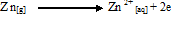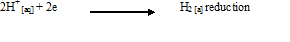The electrons of zinc move through wire to strip and hence hydrogen ixons from the acid take up the electrons and form hydrogen gas bubbles. (bubbles of hydrogen formed on the surface of copper metal) Hence the overall reaction is reddox.

Cathode reaction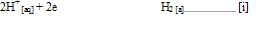Anode reactions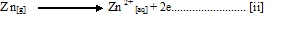Overall reactions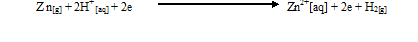Note: copper is the cathode.

Cathode     Electron where reduction occurs

Anode        Electrons are negatively charged they move the negative to positive.

Problems of Galvanic cell

1. During operation the voltage drops as bubbles of hydrogen cover the strip.This is termed polarization.

Solution

The hydrogen bubbles can be removed by

• Brushing them off
• Using an oxidizing chemical.
• Removing the electrode and shake off.

The cell can’t be moved from place to place without spilling content. (Robouk)

Commercial cell

Daniel cell

Is the modified galvanic or voltaic cell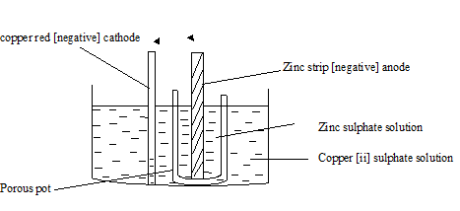Equation of the overall change   -Is got by combining equation for cathode and anode reactions.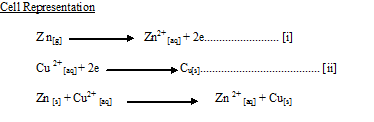Cell representatives

Zn[s] / Zn2+[aq] // Cu 2+[aq]/ Cu[s]

The porous pot

It separates the two electricals. It allows 2 way movements of ions thus completing the circuit.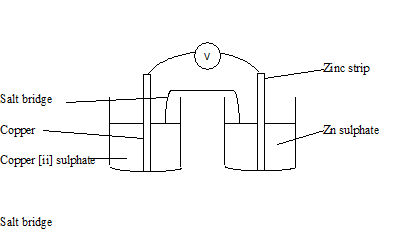It provides electrical contact between the two electrical and problems of the salt bridge Daniel cell.

It can’t be moved from place to place but it does suffer from polarization.

Primary cells

Le clache dry cell

Is the cheapest and most convenient cell used today. It’s portable.

-Used in touches, radios, electric bells.

Summary of reactions which occur

The zinc container is the negative electrode.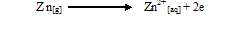The carbon rod is the positive electrode, at which ammonium chloride is reduced to ammonia gas and hydrogen gas.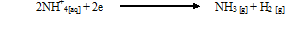• The mixture of powered carbon and manganese (IV) oxide has a 2 fold function.
• Powdered carbon increases the surface are of the carbon rod.
• Manganese (IV) oxide oxidized the hydrogen gas produce to water.
• The ammonia gas formed does not affect efficiency of the lendanche cell

Problems of the leclanche dry cell.

• After used up cannot be recharged
• Some might leak when exeusted

Secondary cells (accumulators)

They are cells which are rechargeable and can use over and over again [backup system cell]

SEE ALL
•YOU